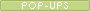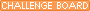mrnelsonalgebra Mr. Nelson Attleboro High SchoolChapter 11 Rational Equations and FunctionsWhat we will be doing in the chapter. 1. Solving Proportions 2. Using equations to solve percent problems 3. Using direct and inverse variation 4. Simplifying rational expressions Section 11.1  - Ratio and Proportion Goal  - Solve proportions Homework  Pg. 646  # 17-22, 35-37, 41, 42 Section 11.2  - Percent Goal  - Use equations to solve percent problems Homework 11.2 Practice A Worksheet Section 11.3  - Direct and Inverse Variation Goal  - Use Direct and Inverse Variation Homework Pg. 459 # 12-16, 21-25, 34, 35,43-46 Section 11.4  - Simplify Rational Expressions Goal   - Simplify rational expressions.        - Use  rational expressions in Geometric Probability Homework Pg. 667 #  10 - 22 Even , 24 - 26, 30, 31 TEST Chapter 11My Quia activities and quizzesRatios and Proportions
https://www.quia.com/jg/135491.html
Solve the ratio, and match the correct valuePop-up Percents
https://www.quia.com/pop/13588.html
Pop the percent, and get the correct answerAlgebraic Jeopardy
https://www.quia.com/cb/13776.html
Play Jeopardy to test your skill on chapter 11